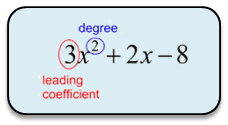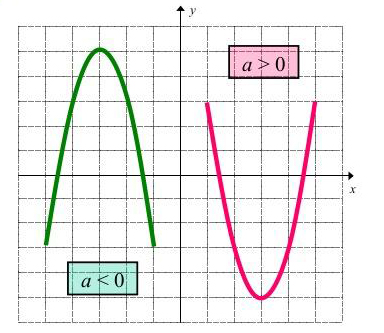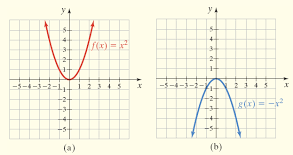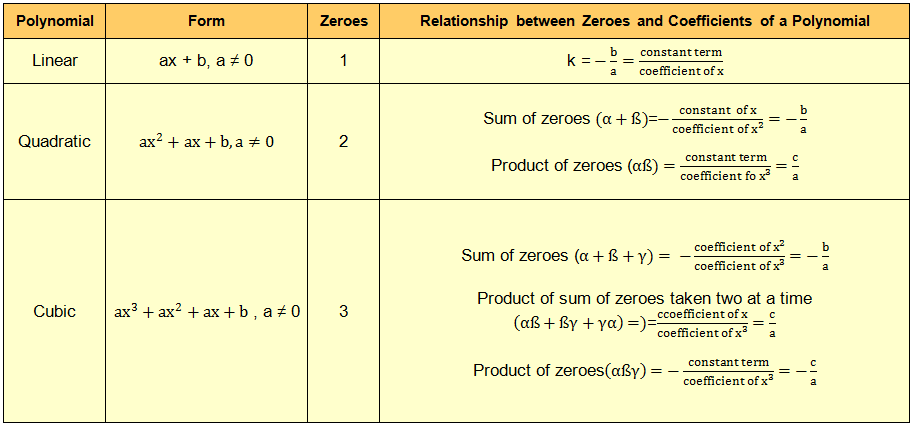×#### Thank you for registering.

One of our academic counsellors will contact you within 1 working day.

Click to Chat

1800-1023-196

+91-120-4616500

CART 0

• 0

MY CART (5)

Use Coupon: CART20 and get 20% off on all online Study Material

ITEM
DETAILS
MRP
DISCOUNT
FINAL PRICE
Total Price: Rs.

There are no items in this cart.
Continue Shopping• Complete JEE Main/Advanced Course and Test Series
• OFFERED PRICE: Rs. 15,900
• View Details

```Revision Notes on Polynomials

A polynomial is an expression consists of constants, variables and exponents. It’s mathematical form is-

a­­­nxn + an-1xn-1 + an-2xn-2 + a2x2 + a1x + a0 = 0

where the (ai)’s are constant

Degree of PolynomialsLet P(y) is a polynomial in y, then the highest #ffffcc power of y in the P(y) will be the degree of polynomial P(y).

Types of Polynomial according to their Degrees

Type of polynomial
Degree
Form

Constant
0
P(x) = a

Linear
1
P(x) = ax + b

2
P(x) = ax2 + ax + b

Cubic
3
P(x) = ax3 + ax2 + ax + b

4
P(x) = ax4 + ax3 + ax2 + ax + b

Value of Polynomial

Let p(y) is a polynomial in y and α could be any real number, then the value calculated after putting the value y = α in p(y) is the final value of p(y) at y = α. This shows that p(y) at y = α is represented by p (α).

Zero of a Polynomial

If the value of p(y) at y = k is 0, that is p (k) = 0 then y = k will be the zero of that polynomial p(y).

Geometrical meaning of the Zeroes of a Polynomial

Zeroes of the polynomials are the x coordinates of the point where the graph of that polynomial intersects the x-axis.

Graph of a Linear PolynomialGraph of a linear polynomial is a straight line which intersects the x-axis at one point only, so a linear polynomial has 1 degree.

Case 1: When the graph cuts the x-axis at the two points than these two points are the two zeroes of that quadratic polynomial.Case 2: When the graph cuts the x-axis at only one point then that particular point is the zero of that quadratic polynomial and the equation is in the form of a perfect squareCase 3: When the graph does  not intersect the x-axis at any point i.e. the graph is either completely above the x-axis or below the x-axis then that quadratic polynomial has no zero as it is not intersecting the x-axis at any point.Hence the quadratic polynomial can have either two zeroes, one zero or no zero. Or you can say that it can have maximum two zero only.

Relationship between Zeroes and Coefficients of a PolynomialDivision Algorithm for Polynomial

If p(x) and g(x) are any two polynomials with g(x) ≠ 0, then we can find polynomials q(x) and r(x) such that

P(x) = g(x) × q(x) + r(x),

where r(x) = 0 or degree of r(x) < degree of g(x).
```### Course Features

• 728 Video Lectures
• Revision Notes
• Previous Year Papers
• Mind Map
• Study Planner
• NCERT Solutions
• Discussion Forum
• Test paper with Video Solution